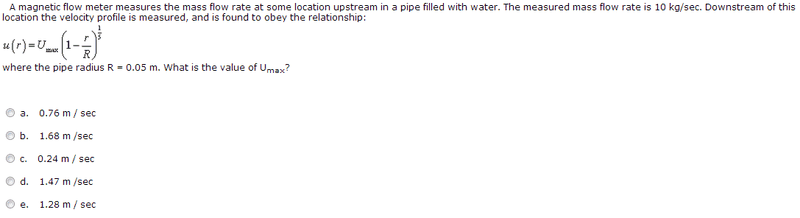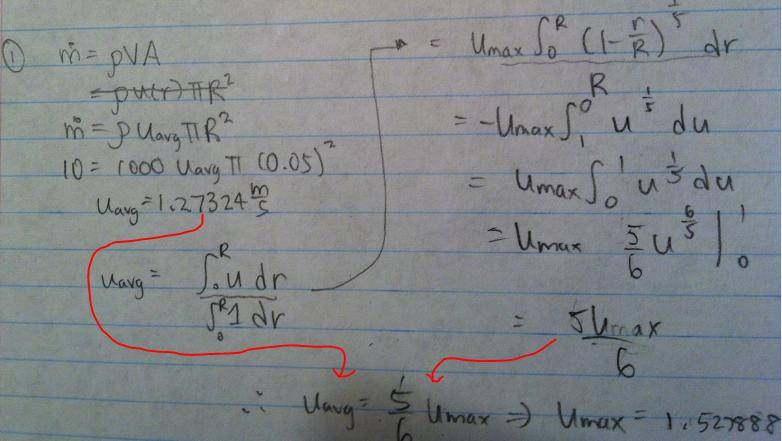# Fluid mechanics - Water flowing through a pipe

## Homework Statement## The Attempt at a Solution

I am interested in why my method is flawed:Alright I know how to do this problem. Essentially you solve mass flow rate = rho * double integral of u dA. HOWEVER, I decided to do this differently. I know that I can solve for the average u from the equation mass flow rate = rho * u_avg * A. I also know that the average u is just the integral of u over the integral of 1. I tried this and I get 1.528 m/s which is clearly not one of the answers. Why does my method not work?

TSny
Homework Helper
Gold Member
In the expression: ##\dot{m} = \rho u_{avg}A##,
##u_{avg}## needs to be the average over the cross-sectional area of the pipe, not the average over the radius.

In the expression: ##\dot{m} = \rho u_{avg}A##,
##u_{avg}## needs to be the average over the cross-sectional area of the pipe, not the average over the radius.

Hmmm, according to the equation doesn't u only depend on r? In other words u is constant for some circular ring r = k?

TSny
Homework Helper
Gold Member
Consider two intervals of radius, one near the center of the pipe and one out at the edge. Suppose the interval dr is the same for both. There are more patches of area in the same interval dr out near the edge. So, the value of the velocity out at the edge contributes more to the average over the cross–section than the value near the center.